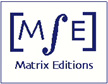Home Contact Us Orders: US Orders: other countries Review Shopping Cart

Serious mathematics, written with the reader in mind.

Matrix Editions

Current Books

Future Books

Errata

Math in literature

Other Books

;

 Vector Calculus, Linear Algebra, and Differential Forms: A Unified Approach, 3rd edition There is now a 4th edition: Vector Calculus 4th editionPreface to the 3rd edition Chapters 1 through 6 of this book cover  the standard topics in multivariate calculus and a first course in linear algebra. The book can also be used for a course in analysis, using the proofs in the appendix.   The organization and selection of material differs from the standard approach in three ways, reflecting the following guiding principles.  First,  we  believe that at this level linear algebra should be more a convenient setting and language for multivariate calculus than a subject in its own right. The guiding principle of this unified approach is that locally, a nonlinear function behaves like its derivative. Thus whenever we have a question about a nonlinear function we will answer it by looking carefully at a linear transformation:  its derivative.  In this approach, everything learned about linear algebra pays off twice: first for understanding linear equations, then as a tool for understanding nonlinear equations. We discuss abstract vector spaces in section 2.6, but the emphasis is on Rn, as we believe that most students find it easiest to move from the concrete to the abstract. Second, we emphasize computationally effective algorithms, and prove theorems by showing that these algorithms work. We feel this better reflects the way this mathematics is used today, in both applied and pure mathematics. Moreover, it can be done with no loss of rigor. For linear equations, row reduction  is the central tool from which everything else follows; we use row reduction to prove all the standard results about dimension and rank.  For nonlinear equations, the  cornerstone is Newton's method, the best and most widely used method for solving nonlinear equations; we use it both as a computational tool and in proving the  inverse and implicit function theorems. We include a section on numerical methods of integration, and we encourage the use of computers  both to reduce tedious calculations and as an aid in visualizing curves and surfaces.   Third, we use differential forms to generalize the fundamental theorem of calculus to higher dimensions. The great conceptual simplifications gained by doing electromagnetism in the language of forms is a central motivation for using forms. We  apply the language of forms to electromagnetism in section 6.9. Complete preface (in pdf) Review of 2nd edition from the Mathematical Association of America Monthly To order (within the United States)